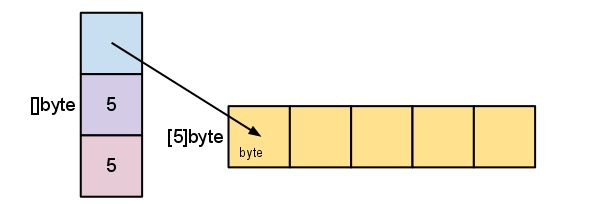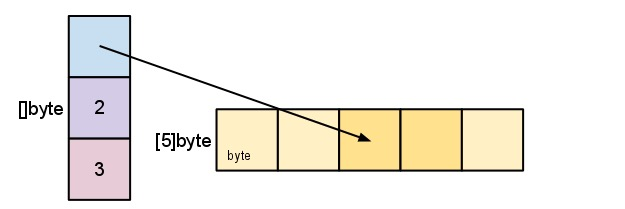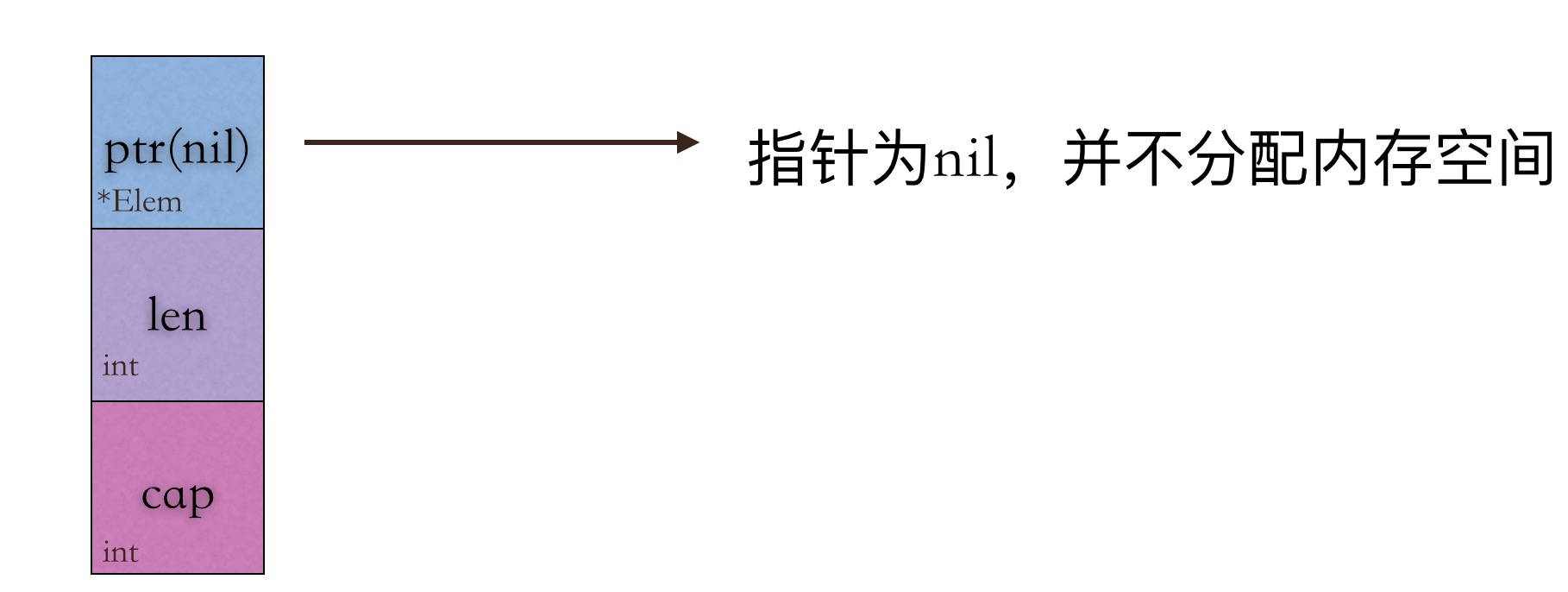Go Array & Slice

数组slice

package main

import (
"fmt"
)

func main() {
var x = make([]float64, 5)
fmt.Println("Capcity:", cap(x), "Length:", len(x))
var y = make([]float64, 5, 10)
fmt.Println("Capcity:", cap(y), "Length:", len(y))
for i := 0; i < len(x); i++ {
x[i] = float64(i)
}
fmt.Println(x)
for i := 0; i < len(y); i++ {
y[i] = float64(i)
}
fmt.Println(y)
}

Capcity: 5 Length: 5
Capcity: 10 Length: 5
[0 1 2 3 4]
[0 1 2 3 4]

package main

import (
"fmt"
)

func main() {
var arr1 = int{1, 2, 3, 4, 5}
var s1 = arr1[2:3]
var s2 = arr1[:3]
var s3 = arr1[2:]
var s4 = arr1[:]
fmt.Println(s1)
fmt.Println(s2)
fmt.Println(s3)
fmt.Println(s4)
}


[1 2 3]
[3 4 5]
[1 2 3 4 5]

package main

import (
"fmt"
)

func main() {
var arr1 = make([]int, 5, 10)
for i := 0; i < len(arr1); i++ {
arr1[i] = i
}
fmt.Println(arr1)
arr1 = append(arr1, 5, 6, 7, 8)
fmt.Println("Capacity:", cap(arr1), "Length:", len(arr1))
fmt.Println(arr1)
}

[0 1 2 3 4]
Capacity: 10 Length: 9
[0 1 2 3 4 5 6 7 8]扩容

package main

import (
"fmt"
)

func main() {
var arr1 = make([]int, 5, 10)
for i := 0; i < len(arr1); i++ {
arr1[i] = i
}
arr1 = append(arr1, 5, 6, 7, 8, 9, 10)
fmt.Println("Capacity:", cap(arr1), "Length:", len(arr1))
fmt.Println(arr1)
}

Capacity: 20 Length: 11
[0 1 2 3 4 5 6 7 8 9 10]

package main

import (
"fmt"
)

func main() {
slice1 := []int{1, 2, 3, 4, 5, 6}
slice2 := make([]int, 5, 10)
copy(slice2, slice1)
fmt.Println(slice1)
fmt.Println(slice2)
}

[1 2 3 4 5 6]
[1 2 3 4 5]

应用

package main

import (
"fmt"
"reflect"
)

func main() {
// index
arrayA := [...]int{0: 1, 2: 1, 3: 4}
fmt.Println(arrayA)

// type
arrayB := [...]int{1, 2, 3}
arrayC := [...]int{1, 2, 3, 4}
fmt.Println(reflect.TypeOf(arrayB))
fmt.Println(reflect.TypeOf(arrayC))
fmt.Println(reflect.TypeOf(arrayB) == reflect.TypeOf(arrayC))

array := int{10, 20, 30, 40}
slice := array[0:2]
fmt.Println(slice)

newSlice := append(append(append(slice, 50), 100), 150)
fmt.Println(newSlice)

newSlice = newSlice + 1
fmt.Println(newSlice)

sliceInt := []int{1, 2, 3, 4, 5, 6}
newSliceInt := append(sliceInt, 1)
fmt.Println(newSliceInt)
}
r := [...]int{99:-1}